/
Features

# Deep Water Animation and Rendering

This GDCE feature introduces a new realtime level-of-detail deep-water animation scheme, which uses many different proven water models. In addition we show how to utilities today’s latest graphic hardware for realistic rendering of oceans. Topics include FFT, surface dynamics, navier-stokes, caustics, godrays, water optics, foam and spray.

In this paper we introduces a new realtime level-of-detail deep-water animation scheme, which uses many different proven water models. In addition we show how to utilities today’s latest graphic hardware for realistic rendering of oceans.

Introduction

This paper introduces a fairly complete animation and rendering model for deep-water. In short the animation model is based on mixing the state-of-the- art water models from the computer graphics literature to suite our need for it to remain realtime. This includes:

• Oceangraphic statistics based surface wave model for ocean waves (FFT)
• Physical correct surface wave model, taking depth into account, for realistic shorelines etc. (Shallow water waves)
• Constraint physical correct wave model for object interaction (Surface waves)
• Full Navier-Stokes simulated bump-map for surface tensions and similar turbulent effects (Navier-Stokes Equations) Our realistic (realtime) rendering of water includes all of the following visual features:
• View dependent water colouring (Colour of water)
• Global reflection/refraction (Reflection/Refraction)
• Local reflection/refraction (Reflection, Refraction)
• Caustics (Caustics) and Godrays (Godrays)
• Foam and spray (Foam, Spray and Bubbles)

Animation

The main philosophy behind our animation is that there is “no single model fitting all needs”. We haven’t tried to make one super model, but instead investigated how to blend between different types and levels of animation. We will first present all the difference models used, and then summaries of how and what we used them for.

FFT
First, we will describe the algorithm we’re using as a core of our sea animation. The algorithm is explained in detail in . This model isn’t based on any physics models, but instead uses statistical models based on observations of the real sea. The method has been used commercially several times, for example for sea animation in the movies Titanic and Waterworld.

In this statistical model of sea, wave height is a random variable of horizontal position and time, h(X,t). It decomposes the wave heightfield into a set of sinus waves with different amplitudes and phases. While the model itself provides us with a tool to generate these amplitudes and phases, we use inverse Fast Fourier Transformation (FFT) as a mean to quickly evaluate the sum.

FFT is a fast version of discrete Fourier transformation, i.e. Fourier transformation that samples the input at regularly placed points. Description of both regular FT and FFT together with it’s interesting properties and working algorithms can be found in , which is also available online.

FFT allows us to quickly evaluate the following sum: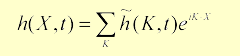Here X is a horizontal position of a point whose height we are evaluating. The wave vector K is a vector pointing in the direction of travel of the given wave, with a magnitude k dependent on the length of the wave (l):

k =2p /l

And the value of h (K,t) is a complex number representing both amplitude and phase of wave K at time t. Because we are using discrete Fourier transformation, there are only a finite number of waves and positions that enters our equations. If s is dimension of the heightfield we animate, and r is the resolution of the grid, then we can write:

K = (kx,kz) = (2pn / s,2pm /s)

where n and m are integers in bounds –r/2 £ n,m < r/2. Note that for FFT, r must be power of two. For rendering the heightfield we need to calculate the gradient of the field to obtain normals. The traditional approach of computing finite difference between nearly placed grid points may be used, but it can be a poor approximation of the slope of waves with small wavelengths. Therefore, if we can afford it (in terms of computational power) it is better to use another FFT, this time evaluating the following sum: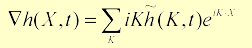Now that we know how to convert field of complex numbers representing wave amplitudes and phases into a heightfield, we need a way to create the amplitudes and phases themselves. Tessendorf  suggests using the Phillips spectrum for wind-driven waves. It is defined by the following equation: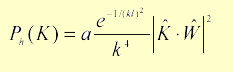In this equation, l = v2 /g the largest possible wave arising from a continuous wind with speed v, g is the gravitational constant, Wˆ is direction of the wind and Kˆ is direction of the wave (i.e. normalized K). a is a numeric constant globally affecting heights of the waves. The last term in the equation (|Kˆ Wˆ|2) eliminates waves moving perpendicular to the wind direction. In this form, the resulting animation contains waves that adhere the wind direction, but move both with and against it, resulting in a lot of meeting waves (and opportunities for splashes and foam creation). If you prefer waves moving in one direction, you can modify this term to eliminate waves that moves opposite to the wind (i.e. the dot product is negative).

Also, to improve convergence properties of the spectrum, we can try to eliminate waves with very small length (w<<l) by multiplying the equation by the following term: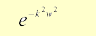There are now two steps we have to do in order to prepare data for FFT – create the amplitudes and phases at time zero, and then animate the field. The first part can be accomplished using the equation: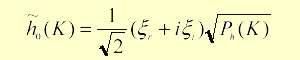Where z r and zi are two independent draws from a Gaussian random number generator with mean 0 and standard deviation 1.

Now, given time t, we can create a field of the frequency amplitudes (independently on previous time, which can be valuable):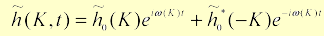Where w is angular frequency of wave k representing the speed at which the wave travels across the surface. You may wonder, what is the source of the right term in this equation? It’s there, because our resulting heights (result of the inverse FFT) are only real numbers (i.e. their imaginary part is equal to zero). It can be shown that for such a function, the following must holds for the amplitudes: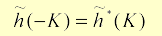where * is the complex conjugate operator 1.

As you may notice, there is one last piece missing in the jigsaw, and that’s value of w for a given wave. Since we are animating deep-water sea, there is a simple relation between w and the corresponding wave-vector K:

w2 (K) = gk

Here g is again the gravitational constant and k is the magnitude of vector K.

There are several modifications to this equation, perhaps the most useful for our purpose is taking depth d into account: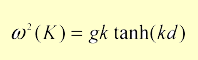Also, if you intend to precalculate the animation, you might try to express each frequency as a multiply of the same basic angular frequency w0 to ensure that the animation loops after a certain time. The results of implementing this set of equations, given above, are a tile of highly realistic sea surface. Given the properties of the FFT it can be seamlessly tiled over and over again. This is a very useful property, even though the tiling can be visible as a repeating pattern. We can improve this by choosing a larger grid, but this obviously comes at a computational expense. Tessendorf  mentions that for the Titanic animation, a grid size of 2048 was used. This is unfortunately too big to be animated in realtime on consumer-class computers. In our experiments we have been using mostly grid size 64, which inverse FFT can be computed quite fast. The size 128 however gives a (subjectively) much better visual result and will probably be the right size in case one are targeting today’s high-end configurations (and the water animation comprise significant part of the whole view).

Choppy Waves
The described algorithm produces nice looking waves, but they all have rounded tops, which suggests nice weather conditions. There is however one modification for making the wave tops sharper and wave bottoms more flat.

Instead of modifying the heightfield directly, we will horizontally displace the positions of the grid points using the equation:

X = X + lD(X,t)

where l is a constant controlling the amount of displacement, and D is the displacement vector computed with FFT: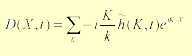The value of l must be carefully chosen – if it’s too big the waves start to intersect them selves, and that certainly breaks the realism. However, detecting this situation seems to be a good way of spawning foam and spray – more on this in chapter 3.7. The difference between the normal waves and the choppy waves modification can be seen in Figure 2-1 and Figure 2-2 respectively.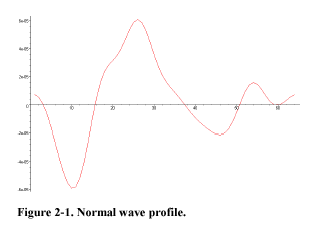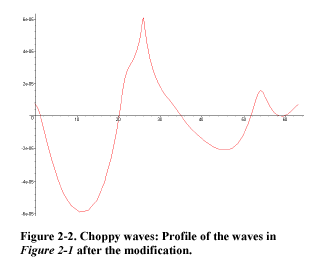Navier-Stokes Equations
In the field of Computational Fluid Dynamics (CFD) the Navier-Stokes Equations (NSE) are know to fully describe the motion of incompressible viscose fluid. In NSE there are three types of forces acting

• Body forces (Fg). These are forces that act on the entire water element. We assume this is gravity only, so Fg= rG, r is density and G is the gravitational force (9.81m/s 2 )
• Pressure forces (Fp). These forces act inwards and normal to the water surface.
• Viscous forces (Fv). These are forces due to friction in the water and acts in all directions on all elements of the water.

The pressure forces are defined as the negative of the gradient of the pressure field of the water elements, i.e.: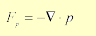Given the fact that water is a Newtonian fluid , i.e. a fluid where the stress is linearly proportional to the strain, the net viscous force (Fv) per unit volume is defined as: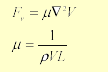Where r is density, V is velocity and L is dimension.

Now that we have all the forces acting in fluids, we will use Newton’s second law (F = mA) to describe the motion: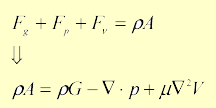Now assuming uniform density we can write the equation as: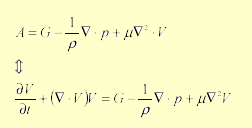This equation conserves the momentum. In addition we need the mass to be conserved: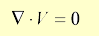These two equations together are referred to as the NSE. Unfortunately the NSE is a set of highly non- linear Partial Differential Equations (PDEs) that’s not easily solved. In the literature there’s many methods for discretizating the PDE both in time (explicit/implicit) and space (Finite Difference, Finite Volume, Finite Element). Going into detail on how to solve the NSE would require a document it self, so instead we will just briefly described what we implemented and how we used the result.

We started by implementing an explicit finite difference scheme on a uniform grid known as the Marker-And-Cell (MAC) method, since this is widely used in earlier works (, , , and ). In short, we divide the solution space into finite cells that holds the velocity and pressure. We then solves Equation 2-9 by finite differences on this grid, for then to enforce Equation 2-10 by an iterative process called Successive Over Relaxation (or one can form a linear system and solve it with for example a Preconditioned Conjugate Gradient method). While solving this is rather simple in closed form, adding boundary conditions and then in particular free-surface conditions is complicated and not well described in the given references. Another problem inherent to finite differences, are stability. Although what’s know as the Courant-Friedrichs- Levy (CFL) conditions for stability can be somewhat enforced by calculating local viscosity and adjusting the time step according to the velocity and the cell size, it gave us unbelievable much pain! We therefore took the time to also implement Jos Stam’s stable solver . Once again it turns out that we can use FFT for solving the closed form, that we will use for the surface details. Stam has recently also released source code for this solver , so one should be able to get up running with this effect quite fast! Once we have a field solved with the NSE, we populate it with particles that are moved according to the bilinear interpolated velocity of the nearest grid elements. These particles will quickly form streamlines (see Figure 2-3) in the field, showing all the turbulent vorticity we expect to see on a tension surface. We then take the finite differences of these particles velocities, and treat them as tangents, for normal calculation. All these normals are then feed into a bump-map, that we apply as real-time surface detail as shown in Figure 2-4.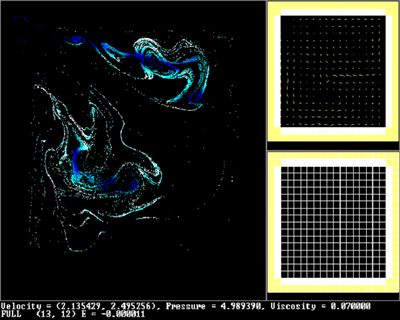Figure 2-3. Our 2D NSE solver showing how the particles forms streamlines in the closed container. The velocity and pressure field is also shown on the right side of the view.

Shallow Water Waves
All of our shallow water simulations are based on  where Kass and Miller use a simplified set of equations to simulate ocean waves. If we look at the horizontal velocity in 2D and assuming, among other things, that the water volume can be described by a height-field we end up with the following set of equations: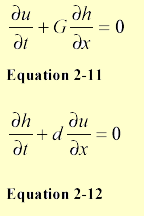Were G is gravity (and other global forces), h is the height of the water surface, d is the depth, and u is the horizontal velocity of a vertical column of water. We can also combine these two equations. Start by differentiate Equation 2-11 with respect to x and Equation 2-12 with respect to t: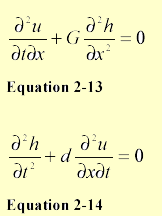Now substituting the partial cross-derivative of Equation 2-14 into Equation 2-13 we end up with: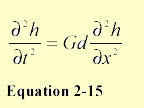Using finite-differences we can discretisate this as: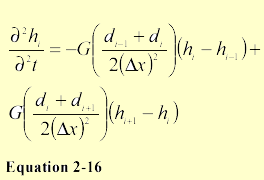Now that we have turned the partial-differential equation into a second order ODE we will solve it using a first-order implicit method. First we will use finite-differences to discretisise the first- and second order time-derivatives of h: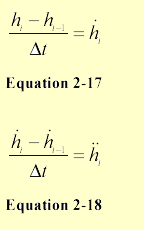We are solving for hi so we will rearrange Equation 2-17 and substitute it into Equation 2-18: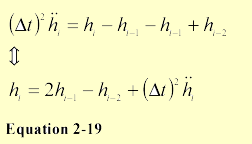And substituting Equation 2-16 into this we get: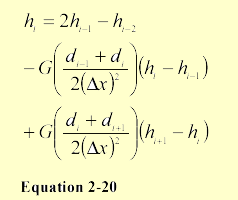The last discretisation done is to treat the depth as constant during iteration, so one ends up with the following linear system: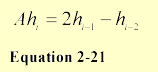Where A is given by: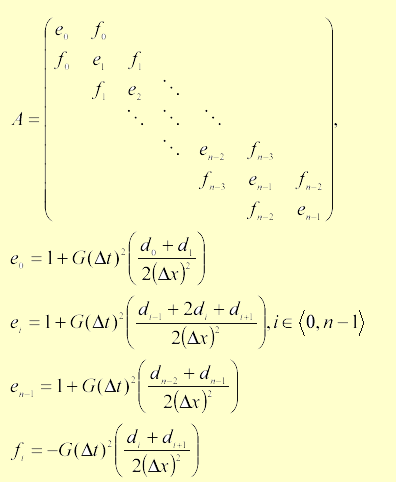Now this matrix gives a symmetric tridiagonal linear system, which can be solved relatively fast, see  for more info. Expanding Equation 2-14 to 3D is done by substituting the partial derivative of h with respect to x with the Laplacian: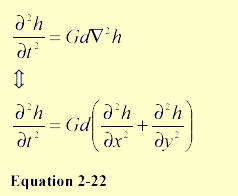And it’s solved exactly as the 2D case simply by splitting it up into two systems - one dependent on x and one on y.

Surface Waves
The last level of animation detail we use is strictly 2D surface waves. If we take our height-field from earlier and constrains the water to a fixed depth, Equation 2-22 reduces to: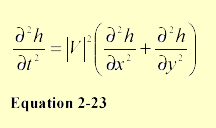Where |V| is the velocity of the wave (across the surface). Let hx,yt be the height of the grid at position x and y at time t, then Equation 2-23 can be discretisised using central differences as :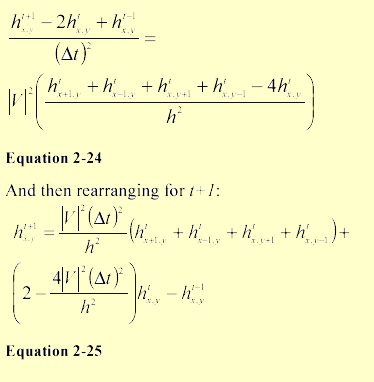As shown in great detail in  this can be animated with just a few arithmetic operation per grid-element

Mixing of the models
Ideally we would like to use NSE for all the water dynamics, but even solutions of order O(n)4 is still too computational expensive for real-time purposes. Instead we decided to use NSE for surface details only, restricting the problem to two dimensions. As mentioned before, the core of our animation is the FFT-water algorithm. This provides us both with large waves, used for the actual geometry, and with small waves optionally used for bump mapping. While the waves generated in this way look very realistic, they have one inherent problem – floating objects cannot interact with the water in any way. This is where the other models come in! We implemented both the shallow water model as described in the section on Shallow water waves and the “traditional” simple surface water as described in the section on surface waves. The shallow water method has several very advanced properties – it takes depth of the surface into account (resulting in waves slowing down and aligning with the coast line) and it can simulate water that floods previously dry areas. However, we didn’t intend to use the model for these large-scale effects and for small waves, around floating objects, the simpler model seems (at least subjectively) to give better results (as well as being a bit easier to control).

For mixing the FFT and physics water, we simply take the geometry from the FFT algorithm and superimpose on it the geometry resulting from the physics model (that’s computed only around floating objects). Although not physically correct, this provides us with the results we desire.

Buoyant Rigid Objects
For adding a rigid object that interacts with the water surface, we need to apply buoyancy to the object and waves to the water surface.

One method for approximating buoyancy is described for example in . As known, according to Archimedes, the force of buoyancy is equivalent to the weight of water displaced by the floating objects. To approximate the displaced volume, we represent the object by a series of patches described by the coordinates of their centre, their area (a) and their normal (N). Then for a given patch (if it’s centre lies in the water), the volume of displaced water can be written as:

V = a(Pwater - Ppatch_center)N

where Pwater is the point on the water surface and Ppatch_center is the position of the centre of the patch. Now, for simplification, we can assume that this force has always direction of the water surface’s normal at the given sampling point. Thus the force applied to the centre of our patch is:

F= rvNwater

where r is the water density. We apply this force to the given point using the standard equation for rigid object physics, as described for example in . There are also two other forces that we should try to simulate. First, floating objects don’t slide freely on the water because of drag. We approximate it for each patch using equation:

Fdrag= ßaV

where ß is constant and V is velocity of the patch centre relative to the water. Also, when object with proper shape moves on the water, it rises out from the water, depending of it’s shape, orientation and velocity (this effect is best seen on boats).

We use the following equation to approximate this effect:

F = -ja(N.V)N

where j is another constant. Note that we use bilinear interpolation of values defined in the grid, to obtain all quantities connected to the water surface, at arbitrary points on the surface.

While this covers the way water affects floating objects, there should also be feedback going in the opposite way. The proper solution would be to take the object into account directly in the physical equations used for animating the water surface, but since values entering these equations don’t represent the complete mixed water anyway, we decided to use another approach. First, for all grid elements touched by any object, we temporally increase the damping factor in the wave model used for object interaction (this creates a wave “shadow” – i.e. place in which waves don’t spread). Secondly, we compute the change in depth of the floating object between the last and current frame, and feed this difference back to the water surface as direct displacement of the surface. With correct scale of this effect, we get both waves from objects that falls into the water and waves formed behind moving objects (such as boats).

Rendering

Reflection/Refraction
Most of the visual effects of water are due to reflections and refractions (more detailed description can be found for example in  and ). When a ray hits the water surface, part of it reflects back to the atmosphere (potentially hitting some object and causing reflective caustics, or hitting the water at other place, or camera), and part of it transmits inside the water volume, scattering (which causes god rays), hitting objects inside the water (causing caustics) or going back into the atmosphere. Thus completely correct lighting would require sophisticated global shading equations and wouldn’t even be close to realtime. We simplify this by only taking first-order rays into account.

Reflection The equation for reflection is well known. For an eye vector E (i.e. the ray from the given point to the eye) and the surface normal N, the reflected ray is:

R=2(E*N)N-E

This ray is then used for lookup in cube-map containing the environment (for ocean typically only the sky).

While the cube-map is ideal for reflecting environment in distance, it’s not very suitable for local reflections (for example boat floating on the water). For this we use a modification of the basic algorithm used for reflections on flat surfaces (described for example in ). We set up the view matrix so that it shows the scene, as it would be reflected from a flat plane placed at height zero, and render the whole scene into a texture. Now if we simple used projective textures, we could render the water surface roughly reflecting the scene above it. To improve the effect, we assume that our whole scene is placed on a plane positioned slightly above the water surface. We intersect the reflected ray with this plane and then compute the intersection of ray between this point and the reflected camera. The resulting point is then fed into the projective texture computations.

Note that when rendering to the texture, we set the camera’s FOV (field of view) slightly higher than one do for the normal camera, because the water surface can reflect more of the scene than a flat plane would.

Refraction We will use Snell’s Law to calculate the refracted ray that we need both for the refracted texture lookup and for the caustics calculations. Snell’s Law is simply: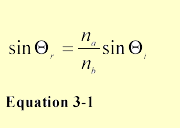Where T i is the angle of incidence (i.e. angle between the view vector and the surface normal), T r is the refracted angle (i.e. between the reflected ray and negate of normal) and na and nb is the index of refractions for the two materials in question. Setting the index of refraction for air and water equal to 1 and 1.333 respectively we can write Equation 3-1 as: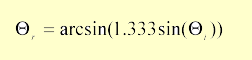While this works perfectly in 2D, use of this equation directly in 3D would be too cumbersome. When using vectors, it can be shown that the refracted ray is described by: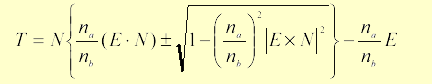Here + sign is used when 0 <·N E . For derivation of this formula, see . With this vector, we are nowready to render the refraction visible on water surface. For the global underwater environment we again use a cube map. For local refractions we use an algorithm very similar to that used for reflections, with only two differences – the scene is rendered into the texture normally, and the plane we’re using for perturbing the texture coordinates is placed below the water surface.

Approximating the Fresnel Term
One of the most important visual aspects of rendering water realistically is due to the Fresnel equation that defines a weight for the blending between the reflection and refraction. Without using the Fresnel term, which defines the amount of reflection according to the incoming light’s angle and the index of refraction of the materials considered, one typical gets a very “plastic look”. From  we have: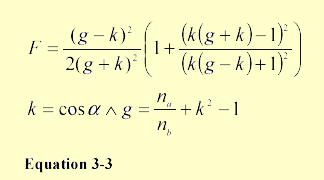Here a is the angle between incoming light and the surface normal and na and nb is the coefficients from Snell’s law (Equation 3-2). Since we use an index of 1.333 g only depends on k, so it’s possible to precalculate this and store it in a one-dimensional texture . Another possibility is to approximate Equation 3-3 with a simpler function so we can calculate it directly with the CPU or on the GPU using vertex-/pixel-shaders. In the implementation of  they approximate this simply by a linear function that we didn’t find adequate. Instead by experimentations we found out that reciprocal of different powers gives a very good approximation.

In Figure 3-1 we can see the error-plot of a few different powers, and in Figure 3-2 we see our chosen power compared against Equation 3-3.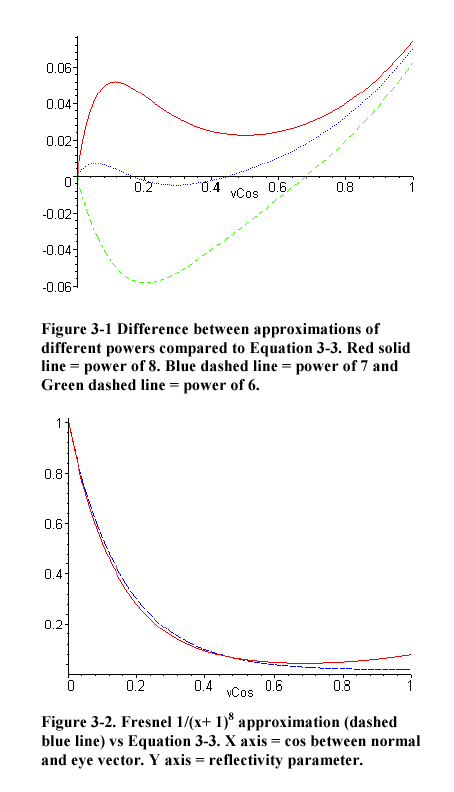Color of Water
In chapter 3.1.2 we have described how to render refractions on the water surface. It should however be noted that for deep water, only local refractions should be rendered since one cannot see the sea bottom or any other deeply placed objects (and even the local refractions should be rendered with some kind of fogging). The water itself however has colour that depends on the incident ray direction, the viewing direction and the properties of the water matter itself. To remedy for this effect we take the equations presented in , that describes light scattering and absorption in water, and modify them as described shortly. If we don’t take any waves into account (i.e. we treat the water surface as a flat plane) and ignore effects like Godrays, we obtain closed formulas for the watercolour depending only on the viewing angle. This colour is then precalculated for all directions and stored in a cube-map, which is used in exactly the same way as the cube-map for the refracted environment was.

Thanks to that we get darker blue water when looking into depth and brighter greenish colour when looking at the waves, as shown in Figure 3-3.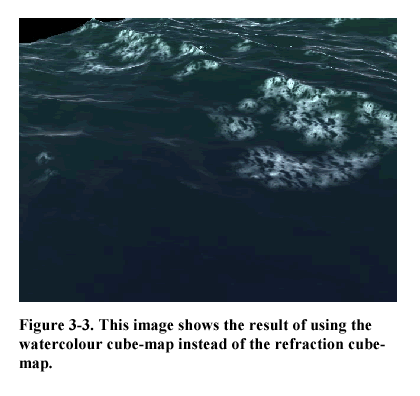Using Bump-Mapping to Reduce Geometry
In addition to using traditional Level-Of-Detail (LOD) methods for reducing our dense mesh, we can place the highest frequencies from the FFT directly into a bump-map. With the per pixel bump-mapping capabilities of new hardware, one can render with an extremely coarse grid-size and still maintain a hi image quality as shown in Figure 3-4 with it’s wireframe shown in Figure 3-5.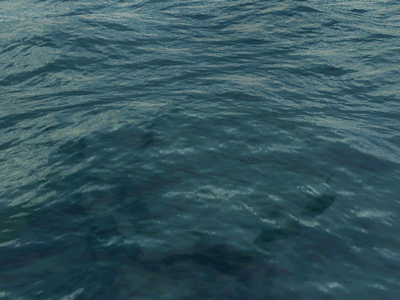Figure 3-4. Shallow water rendered with a real-time updated bump-map. Due to the refraction one can see contours of the mountain below.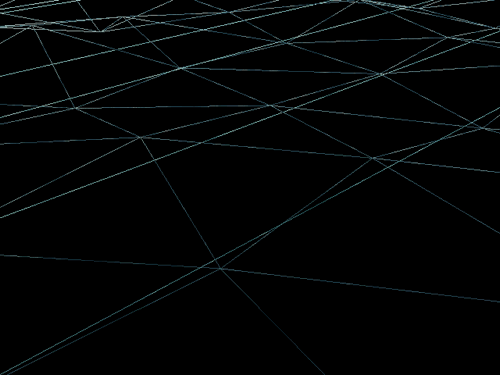Figure 3-5. Wireframe of the mesh used to render the image in Figure 3-4. Please note that the crossing lines are due to the degenerated trianglestips.

Caustics
Caustics are beautiful light sinuous shifting patterns due to sunlight transmitted from the specular water surface. Caustics are a typical indirect lighting effect and are generally very hard to do in realtime. Luckily we can optimise the problem by only considering first order rays (i.e. only one specular-diffuse transmission) and by assuming the receiving diffuse surface is at a constant depth. Now given these, visual acceptable, constraints we use a light beam-tracing scheme described by Watt&Watt . For each specular triangle (i.e. our water surface) we create a light beam by calculating refracted rays for each vertex using Snell’s law (Equation 3-1) with the vertex’s normal (Nv) and the light-vector (i.e. vector from sun to the vertex) (L) as arguments. These light beams are then intersected against the xz-plane (our sea-bottom) at a given constant y- depth. See Figure 3-6 for an illustration of this method. Each of these beams will then diverge or converge on to the plane, so we need to describe their intensity. In  they use the following: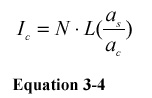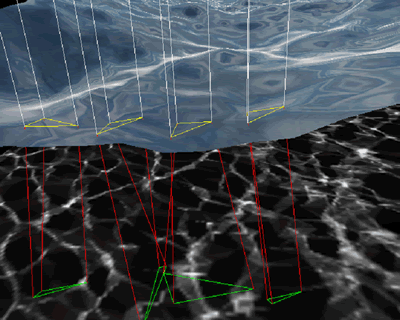Figure 3-4. Please note that the crossing lines Figure 3-6. Four sample triangles for caustics computation.

Where N is the normal of the triangle, L as defined earlier, as is the area of the specular surface (i.e. triangle at the water surface) and ac is the area of the caustic surface (i.e. triangle after intersecting with the xz-plane). Since we know that the entire water surface is refracted as light beams we can simply create one huge degenerated 5 triangle-strip for the caustic mesh, and update the position and intensities of this mesh’ vertices as described.

Unfortunately although the FFT water surface tiles, the resulting caustics pattern does not, because we use only one tile of the surface in the computations. Since calculating the caustics takes considerable time we can’t afford to calculate it for the entire ocean, so we need a way to make it “tileable”. We solve this by blitting parts of the resulting caustic texture nine times, one for each directions, from a large caustic texture. Each part is added to the middle “cut out” which we use as the final caustics texture. This process is illustrated in Figure 3-7 with the result shown in Figure 3-8. A nice side effect of this process is that we can use the multi- texturing capabilities of today’s hardware to do Anti-Aliasing at the same time. We simply set up four passes of the same texture and perturblate the coordinates of each pass slightly to simulate the effect of a 2x2 super-sampling. This is in our opinion needed, since the caustics patterns has a lot of details that quickly aliases if the specular surface isn’t dense enough to represent the pattern properly. On the other hand we could of course use the other passes to reduce the number of blits.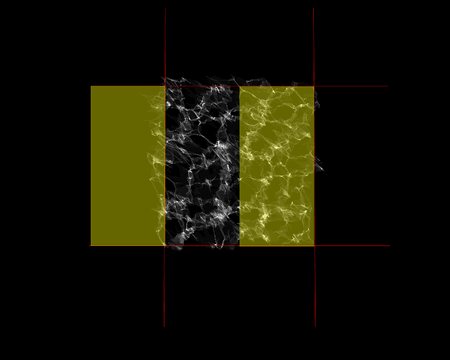Figure 3-7. The left part of 1024x1024 caustics texture is added to the right half of the inner 256x256 part of the image. A similar process is done for the eight other pieces.

Since the caustics patterns changes rapidly with depth, as seen in Figure 3-9, we use the camera’s bounding box and previous depth to decide an average depth to use.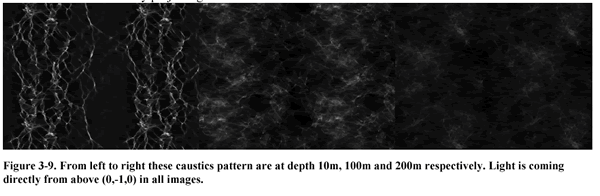For applying this texture to objects underwater, we need a way to calculate the texture-coordinates into the caustics texture. Given the sun’s ray direction and the position of a triangle, we compute it’s texture’s UV coordinates by projecting the texture from the height of the water in the direction of the ray (note that because this works as a parallel projection, we don’t even have to use projective textures here). In addition we compute the dot product between the surface’s normal and the inverted ray direction to obtain the intensity of the applied texture (we use this as alpha then). The same algorithm can be used to create reflective caustics on objects above water.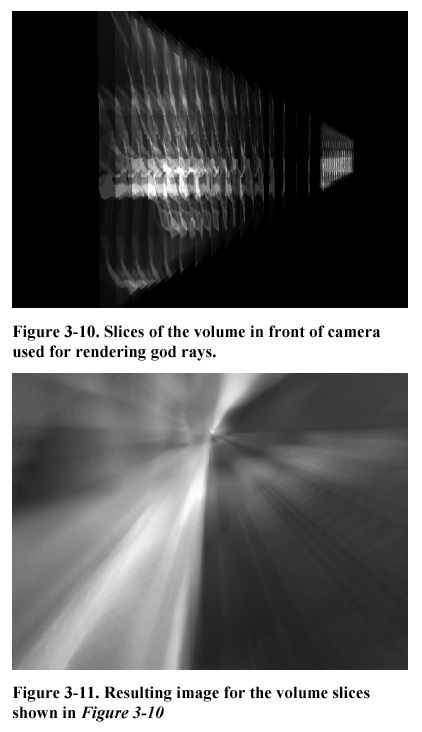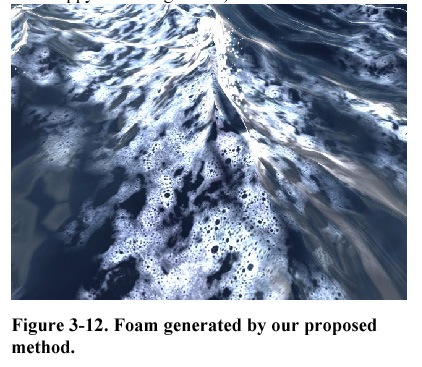Godrays
In the last section, we described how the light causes caustics by the water surface focusing and defocusing light rays. However as the rays pass the water matter, they scatter from small particles floating in the water (plankton, dirt), making them visible and causing streaks of light known as Godrays. Rendering this phenomenon correctly would require volumetric rendering. However if we don’t insist on absolute correctness, preferring the visual look of the result, we can use a quite simple algorithm to create relatively convincing pictures. We already have the caustics texture, which represents shape and positions of the individual ray streaks (even though only as a slice at given depth). If we define this slice to represent the light intensity for the whole volume, we can render it using techniques for volumetric rendering.

Given position of our camera, we create several (in our experiments 32) slices of the volume as seen in Figure 3-10. We then render them into the completed scene with additive alpha-blending (and writes to zbuffer disabled).

Because this method shows visible artefacts – revealing the low sampling, we use a non-uniform distribution of the samples. We use high density in front of camera – these samples are responsible for the smooth look of the result and for bright spots where they should be. The lower density samples further away from the camera ensure that the rays extend into distance.

Since increasing the number of rendering passes considerably slows down the whole process, we can use the multitexturing capabilities of graphics hardware to increase the number of samples as suggested in . So even if we render just one slice, we apply to it four textures at once as if they represented subsequent samples of the volume. In this way we obtain 128 samples on the GeForce3, which gives us smooth enough pictures in most cases (as seen in Figure 3-11).

Note that we can “skew” the volume, resulting from repeating our caustics texture, in any way to simulate rays going from a given direction (according to position of the sun). An additional improvement (which we didn’t implement) would be to use shadow buffer to take shadows cast by objects in water into account.

Foam, Spray and Bubbles
Whenever the water surface is violent enough or the water meeting obstacles, we should see foam resulting from the breaking waves. Probably the best way to render this would be to use particle system, but this would be quite costly for our purposes. Instead, we take advantage from the fact that the foam always lies on the water surface and render is as another texture layer on top of it. In our implementation, each vertex of the grid has assigned an “amount of foam” to it. Then, when rendering the surface, we use this amount as transparency for the foam texture stretched over the whole surface (the texture itself is rendered with additive blending). Now the only thing left to solve is spawning of the foam itself. Here we use modification of the algorithm suggested in . For a given vertex and its two neighbours (in x and z direction) we compute the difference between their slopes. If you remember the way we animated choppy waves, the displacement used does in fact represent how close these points get. Now if the computed difference is less than a chosen (negative) limit, we increase the foam amount for the given vertex by a small number. Otherwise, we decrease its current foam amount (causing the foam already existing to fade away). In this way we get foam spawning near tops of big choppy (and possibly meeting) waves. See Figure 3-12 for a typical result of the foam generation.

It is important to note that even though the alpha factor of the foam texture is limited to the range [0,1], this is not true for the foam amount (that can be more then one, but should be still limited). Also, when we detect a foam-producing point, we shouldn’t set its foam amount immediately to maximum – the vertex is likely to spawn foam the next few frames as well, and increasing the foam amount slowly gives a better visual result. Limitations of this technique are quite obvious – the rendered foam looks quite similar at different places (since it’s just a repeated texture, not an uniquely generated pattern), and it doesn’t move on the water surface according to it’s slope (though one might get the impression that this is happening when using the choppy waves algorithm).

Particle System
When water collides against obstacles we generate spray of water using a particle system with simple Newtonian dynamics, see . Each particle is given an initial velocity taken directly from the water-surface’s velocity, at the spawning position, with added turbulence. It’s then updated according to gravity, wind and other global forces thereafter. Rendering of the particles are done with a mixture of alpha-transparency and additive-alpha sprites. See Figure 3-13 for a screen shot of this effect. The particle system is also used for drawing bobbles from objects dropped into the water. For this effect we simply move the bobbles on a sinus path around the buoyancy vector up to the surface were they are killed.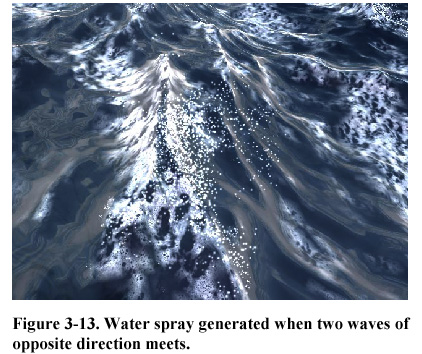Implementation Details

We implemented the algorithms described in this paper on a PC platform with windows. Both the FFT-based and physical-based animations were realized for grids with 64x64 elements. Two FFTs were required for the animation, one complete complex complex for the surface slope (that is later used either for the choppy waves or for surface normals) and one complex real for surface height. Our first implementation used routines from , but later we replaced them by faster routines from the Intel . Math Kernel Library. Rendering is implemented in DirectX 8.1 using nVidia’s GeForce3 hardware for rendering. While the basic computations (heights, normals, foam etc.) is done only once for a single water tile (that can be repeated all over the place), many other computations depends on the viewer position (we use local viewer everywhere) and thus had to be done separately for each tile. This offers perfect opportunity for the use of vertex shaders, offloading the burden of those computations from CPU. Some of these effects (especially per-pixel bump-mapping) requires the use of pixel shaders as well, but in general most of the algorithms described here should be possible on DirectX7 class hardware.

Summary and Future Extensions

We have presented a new scheme for deep-water animation and rendering. It’s main contributions on the animation side is the blending of proven methods for realistic object/ocean interaction. On the rendering side we have presented a new method for foam rendering and shown clever use of the new 3D graphic cards features to reach new levels of (realtime) realism. There are many extensions, to the current implementations, that we want to try out in the future. First of all we are not to impressed by our Phong shaded water shimmering. We believe this is mainly because of too low contrast in the final image. Contrast enhancement can probably be realised by using Hi-Dynamic Range Images (HDRI), as described in . We also want to try prefiltering of the environment-map  to approach the BRDF shading of water. When it comes to animation, there’s so much cool stuff out there to follow up! Foremost we are trying to get the ocean sinus model from  to work with our system…breaking waves next?

Acknowledgements
We would like to thank Richard Lee for implementing the choppy waves modification and “forcing” us to add light shimmering. Super-hero-star to Tore Blystad and Christian Morgan Enger for their excellent demo artwork, and a final thank to Mads Staff Jensen for the slide illustrations!

References
 Alan Watt and Mark Watt. “Advanced animation
and rendering techniques”. ISBN 0-201-54412-1
 Jerry Tessendorf. “Simulating Ocean Water”.
SIGGRAPH 2001 Course notes.
http://home1.gte.net/tssndrf/index.html.
 Miguel Gomez. “Interactive Simulation of Water
Surfaces”. Game Programming Gems. ISBN 1-58450-
049-2.
Reflection Using Glossy Prefiltering and the Fresnel
term”. Game Programming Gems. ISBN 1-58450-
049-2.
 Alex Vlachos and Jason L.Mitchell. “Refraction
Mapping for Liquids in Containers.” Game
Programming Gems. ISBN 1-58450-049-2.
 Press, Teukolsky, Vetterling, Flannery. “Numerical
Recipes in C, The Art of Scientific Computing”.
Second edition. Cambridge University Press. ISBN
0-521-43108-5.
 Jim X. Chen, Niels da Vitoria Lobo, Charles E.
Hughes and J.Michael Moshell. “Real-Time Fluid
Simulation in a Dynamic Virtual Environment”.
IEEE Computer Graphics and Application. May-June
1997, pp.52-61.
 Nick Foster and Dimitri Metaxas. “Realistic
Animation of Liquids”. Graphical Models and Image
Processing, 58(5), 1996, pp.471-483.
 Nick Foster and Dimitri Metaxas. “Controlling Fluid
Animation”. Proceeding of the Computer Graphics
International (CGI’97).
 Nick Foster and Dimitri Metaxas. “Modeling the
Motion of a Hot, Turbulent Gas”. Computer
Graphics Proceeding, Annual Conference Series,
1997, pp. 181-188.
 Jos Stam. “Stable Fluids”. SIGGRAPH’99
Proceedings.
 C.Rezk-Salama, K.Engel, M.Bauer, G.Greiner,
T.Ertl. “Interactive Volume Rendering on Standard
PC Graphics Hardware Using Multi-Textures And
Multi-Stage Rasterization”
 David Baraff, Andrew Witkin. “Physically Based
Modeling” SIGGRAPH 98 course notes.
 Mark J.Kilgard. “Improving Shadows and
Reflections via the Stencil Buffer”, nVidia white
paper.
 Foley, van Dam, Feiner and Huges. “Computer
Graphics. Principles and Practice.” ISBN 0-201-
84840-6.
 Tomoyuki Nishita, Eihac hiro Nakamae. “Method of
Displaying Optical Effects within Water using
Accumulation Buffer”
 Michael Kass and Gavin Miller. “Rapid, Stable Fluid
Dynamics for Computer Graphics”. Computer
Graphics, Volume 24, Number 4, August 1990.
 Joe Stam. “A Simple Fluid Solver based on the
FFT”. Journal of Graphics Tools.
http://reality.sgi.com/jstam_sea/Research/pub.html
 Hugh D. Young. “University Physics. Eighth
edition”. ISBN 0-201-52690-5.
 Lasse Staff Jensen. ”Game Physics. Part I:
Unconstraint Rigid Body Motion”.
 Wolfgang Heidrich. “Environment Maps And Their
Application”.
 Jonathan Cohen, Chris Tchou, Tim Hawkins and
Paul Debevec. “Real-time High Dynamic Range
Texture Mapping.”. Eurographics Rendering
Workshop 2001.
 Alain Fournier and William T. Reeves. “A simple
model of Ocean waves”. SIGGRAPH 1986
Proceedings.

______________________________________________________

### Latest Jobs

#### IO Interactive

Hybrid (Malmö, Sweden)
3.02.23
Gameplay Director (Project Fantasy)

#### Arizona State University

Los Angeles, CA, USA
2.27.23
Assistant Professor of XR Technologies

#### IO Interactive

Hybrid (Copenhagen, Denmark)
3.02.23
Animation Tech Programmer

#### Purdue University

West Lafayette, IN, USA
3.02.23
Assistant Professor in Game Design and Development
More Jobs

#### Game Developer Job Board

Browse open positions across the game industry or recruit new talent for your studio